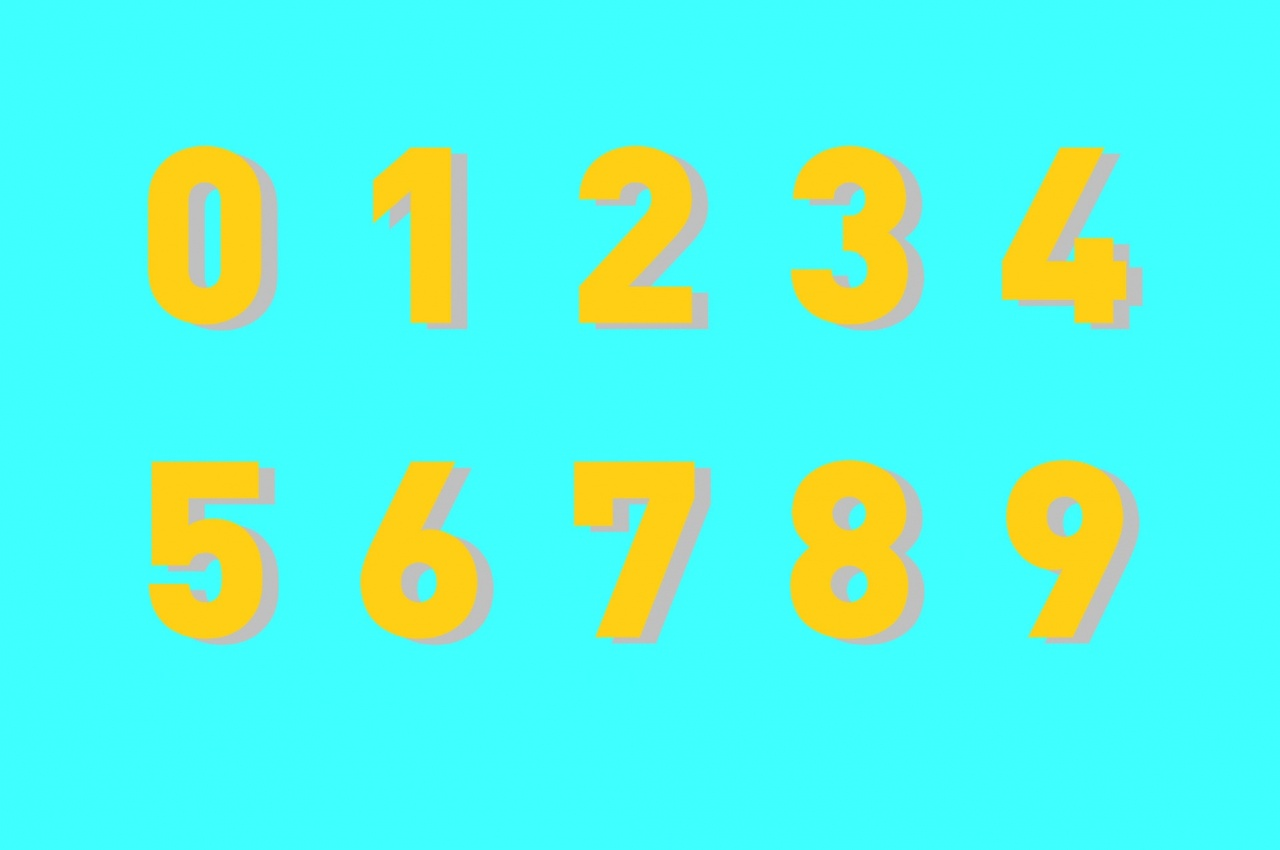👋🏼 Var med och förbättra Skolbanken med oss på Unikum. Svara på formuläret här

# Integers

Skapad 2021-05-15 09:19 i Rödabergsskolan Stockholm Grundskolor
Grundskola 4 – 6 MatematikFinally we can count backwards past zero on the number line! This unit is about positive and negative numbers. How to identify them, calculate them, add them, subtract them, multiply and divide them. Just remember to watch the signs!

### Innehåll

Lesson Content

• Scott Foresman Year 6 Textbook

• Scott Foresman Year 6 Practice Masters Workbook

• NOMP

• All work is done individually, but you can of course help each other.

Goals

• Understand that there are numbers less than zero (negative numbers)

• Understand how to use a number line to compare and add integers

• Understand that positive and negative numbers can also be fractions and decimals

• Understand that subtracting an integer is the same as adding its opposite

• Understand that when you multiply and divide integers, the product can be positive, negative or zero.

• Understand how to solve equations with integers.

Key Terms

• Opposites

• Integers

• Absolute Value

• Rational numbers

• nomp.se

• Quality of in class work

• Quiz at the end of the unit.

### Kopplingar till läroplanen

• Syfte
• formulera och lösa problem med hjälp av matematik samt värdera valda strategier och metoder,
Ma
• välja och använda lämpliga matematiska metoder för att göra beräkningar och lösa rutinuppgifter,
Ma
• Centralt innehåll
• Rationella tal och deras egenskaper.
Ma  4-6
• Positionssystemet för tal i decimalform.
Ma  4-6
• Tal i bråk- och decimalform och deras användning i vardagliga situationer.
Ma  4-6
• Metoder för enkel ekvationslösning.
Ma  4-6

### Ma Math Year 4-6 Simplified (English)

E Level
C Level
A Level
Problem Solving
Pupils can solve simple problems in familiar situations in a basically functional way by choosing and applying strategies and methods with some adaptation to the type of problem.
Pupils can solve simple problems in familiar situations in a relatively well functioning way by choosing and using strategies and methods with relatively good adaptation to type of problem.
Pupils can solve simple problems in familiar situations in a well functioning way by choosing and using strategies and methods with good adaptation to the type of problem.
Concept
Pupils have basic knowledge of mathematical concepts and show this by using them in familiar contexts in a basically functional way. Pupils can also describe different concepts using mathematical forms of expression in a basically functional way. In the descriptions, pupils can switch between different forms of expression and also apply simple reasoning over how the concepts relate to each other.
Pupils have good knowledge of mathematical concepts and show this by using them in familiar contexts in a relatively well functioning way.Pupils can also describe different concepts using mathematical forms of expression in a relatively well functioning way. In the descriptions, pupils can switch between different forms of expression and also apply developed reasoning over how the concepts relate to each other.
Pupils have very good knowledge of mathematical concepts and show this by using them in new contexts in a well functioning way. Pupils can also describe different concepts using mathematical forms of expression in a well functioning way.n the descriptions, pupils can switch between different forms of expression and also apply well developed reasoning over how the concepts relate to each other.
Method
Pupils can choose and apply basically functional mathematical methods with some adaptation to the context to carry out simple calculations and solve simple routine tasks in arithmetic, algebra, geometry, probability, statistics and also relationships and change with satisfactory results.
Pupils can choose and apply appropriate mathematical methods with relatively good adaptation to the context to carry out simple calculations and solve simple routine tasks in arithmetic, algebra, geometry, probability, statistics, and also relationships and change with good results.
Pupils can choose and apply appropriate and effective mathematical methods with good adaptation to the context to carry out simple calculations, and solve simple routine tasks in arithmetic, algebra, geometry, probability, statistics, and also relationships and change with very good results
Communication
Pupils can account for and discuss their approaches in a basically functional way and use diagrams, symbols, tables, graphs and other mathematical forms of expression with some adaptation to the context.
Pupils can account for and discuss their approaches in an appropriate way and use diagrams, symbols, tables, graphs and other mathematical forms of expression with relatively good adaptation to the context.
Pupils can account for and discuss their approaches in an appropriate and effective way and use diagrams, symbols, tables, graphs and other mathematical forms of expression with good adaptation to the context.
Rationale
Pupils describe their approach in a basically functional way and applysimple and to some extent informed reasoning about the plausibility of results in relation to the problem situation, and can also contribute to making some proposals on alternative approaches. In their accounts and discussions, pupils can apply and follow mathematical reasoning by putting questions, putting forward and responding to mathematical arguments in a way which to some extent takes the reasoning forward.
Pupils describe their approach in a relatively well functioning way and apply developed and relatively well informed reasoning about the plausibility of results in relation to the problem situation, and can make some proposals on alternative approaches. In their accounts and discussions, pupils apply and follow mathematical reasoning by putting questions, putting forward and responding to mathematical arguments in a way which takes the reasoning forward.
Pupils describe their approach in a well functioning way, and apply well developed and well informed reasoning about the plausibility of results in relation to the problem situation, and can make proposals on alternative approaches.In their accounts and discussions, pupils can apply and follow mathematical reasoning by putting questions, putting forward and responding to mathematical arguments in a way which takes the reasoning forward and deepens or broadens them.